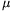Mathematical and Physical Journal
for High Schools
Issued by the MATFUND Foundation
 Already signed up? New to KöMaL?

#Problem P. 4352. (May 2011)

P. 4352. A rough inclined plane of height h=2 m, and of angle of elevation 20o consists of two parts. The two parts have the same length but the friction is different on them. At the bottom of the slope a small body was pushed, and it slid to the top of the plane and then it slid back and it just stopped at the end of the slope. a) What was the initial speed of the small body at the bottom of the slope? b) What is the coefficient of friction along the bottom part of the slope if the coefficient of friction at the top is2=0.3?

(4 pont)

Deadline expired on June 10, 2011.

Sorry, the solution is available only in Hungarian. Google translation

Megoldás. $\displaystyle a)$ 8,9 m/s.

$\displaystyle b)$ $\displaystyle \mu_1=0{,}43.$

### Statistics:

 96 students sent a solution. 4 points: 71 students. 3 points: 9 students. 2 points: 13 students. 1 point: 2 students. Unfair, not evaluated: 1 solutions.

Problems in Physics of KöMaL, May 2011